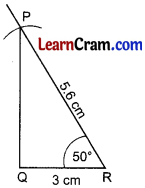# DAV Class 7 Maths Chapter 10 Worksheet 2 Solutions

The DAV Class 7 Maths Solution and DAV Class 7 Maths Chapter 10 Worksheet 2 Solutions of Construction of Triangles offer comprehensive answers to textbook questions.

## DAV Class 7 Maths Ch 10 WS 2 Solutions

Question 1.
Construct a ∆AOB given that OA = 5cm, OB = 4.5 cm and ∠AOB = 50°.
Steps of Construction:1. Draw OB = 4.5 cm.
2. Draw an angle of 50° at O.
3. Cut OA = 5 cm
4. Join AB.
5. Triangle AOB is the required triangle.

Question 2.
Draw ∆ABC in which ∠A = 120° and AB = AC = 3 cm. Draw the bisector of angle A.
Steps of Construction:1. Draw AB = 3 cm.
2. Draw an angle of 120° at A.
3. Cut AC = 3 cm.
4. Join CB.
5. Draw AY as the bisector of ∠A.
6. ∆ABC is the required triangle.Question 3.
Construct a ∆ABC in which ∠B = 90° and AB = 4 cm and BC = 3 cm.
Steps of Construction:1. Draw BC = 3 cm.
2. Draw an angle of 90° at B.
3. Cut BA = 4 cm.
4. Join AC.
5. ∆ABC is the required triangle.

Question 4.
Draw a triangle PQR in which QR = 4 cm, PQ = 3 cm and ∠Q = 45°.Steps of Constructions:

1. Draw PQ = 3 cm.
2. Draw an angle of 45° at Q.
3. Cut QR = 4 cm.
4. Join PR.
5. ∆PQR is the required triangle.Question 5.
Construct a triangle PQR in which PR = 5.6 cm, QR = 3 cm and ∠R = 50°.
Solution:
Steps of Construction:• Draw QR = 3cm.
• Draw an angle of 50° at R.
• Cut RP = 5.6 cm Join PQ.
• ∆PQR is the required triangle.